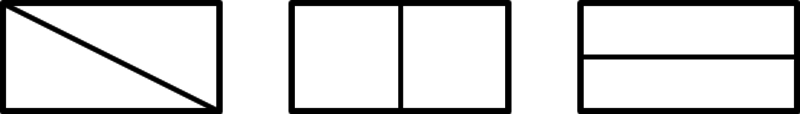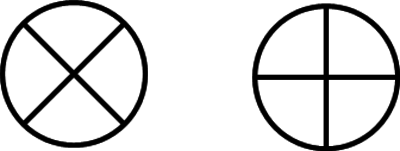# Equal Shares

Alignments to Content Standards: 1.G.A.3

Materials

• Paper cut outs of rectangles, circles, and squares

• Blank paper

Actions

Part One:

1. Give each pair of students a square and ask, “How can you share the square equally so that you and your partner get the same size piece?”

2. Ask students to fold the paper to show how they could get two equal parts.

3. Call on student volunteers to share, asking the class as each example is displayed, “Is the paper shared equally?  Will each person get the same size piece?  How do you know?”

4. Create a chart, showing some of the ways students folded the square to make two equal parts.  Students may fold vertically, horizontally, or diagonally.

5. Tell students, “There are two equal parts.”  Ask students, “What can we call each part of the rectangle?”  Elicit student thinking, building the understanding that each piece is one of two equal parts, or half of the rectangle.  The standard calls for students to "describe the shares using the words halves, fourths, and quarters, and use the phrases half of, fourth of, and quarter of."  Helping students develop this language is critical.  If students don't come up with this language, it's important to introduce it.  After introducing the terms "half" and "half of" in this case, adding labels to the chart will help anchor the language.

You may repeat this with other shapes (circle, rectangle) or increase the number of students sharing the paper shape from 2 to 4. If you repeat with a rectangle some students may a scissors as scaffolding to cut the rectangle instead of folding when they divide the rectangle diagonally.

Part Two:

1. Pose the problem to students:  "If you and three friends want to share a cake so that you each get the same amount, how can you much can each person have?"  Ask questions to ensure students understand the context and problem being posed.  Questions might include:

• What is the story about?
• How many people are sharing the cake?
• What does it mean for each person to get the same amount?

Provide students with tools such as blank paper and/or paper shapes to solve the problem.  The cake context was selected to allow students to explore with multiple shapes as cakes may be round, square, or rectangular in shape.

1. As students solve the problem, monitor their progress looking for students that partition a shape into four equal shares.

2. Conduct a share out, showing several different student solutions that show a shape partitioned into four equal parts.  It may be helpful to consider the features of each solution being shared by posing questions that get at student thinking and the essential mathematical ideas.

The teacher might ask questions such as:

• How many people are sharing the cake?

• What does the picture represent?  What is the circle?  What is the square?  What is the rectangle?

• How is ___'s picture similar or different than ____'s?

• How much cake does each person get?

• Some people represented the cake with rectangles and others with squares or circles, did that change the amount of cake each person gets?  Why or why not?

• What can we call these parts?  How can we label them?

• Why do the parts need to be equal?

## IM Commentary

This purpose of this task is for students to understand how to partition shapes into equal pieces.  This task starts students with concrete representations of the shapes that they can fold and cut, so that later they will understand more abstract representations like diagrams and symbols. Part one provides students with opportunities to manipulate paper shapes, folding them to create equal parts.  Students start with a square so that vertical, horizontal, and diagonal folds all match the two sides exactly, this makes it easy to see what is meant by “equal parts in this case. Rectangles pose a greater challenge, because the diagonal fold does not match the two halves up exactly. The teacher should lead a discussion about how we know the two halves are equal by cutting them apart and showing they match up exactly (or providing students with scissors and allowing them to do this for themselves). The idea of “equal parts” with shape is actually very subtle; in grade 1, students only look at congruent figures as equal. Later they can talk about equal area even when shapes aren’t congruent; students begin to tackle these issues in Grade 3 and beyond.

Through a class discussion, students will understand that shapes can be partitioned in many different ways and that there are conventions for naming those parts. The task intentionally begins with partitioning a shape into two equal parts to build on students intuitive knowledge and experience working with halves.  Students may be familiar with the term half but think of it as two parts rather without understanding that the parts must be equal in some sense.  Developing student language is critical component of this task, the standard calls for students to "describe the shares using the words halves, fourths, and quarters, and use the phrases half of, fourth of, and quarter of."

Part one can be extended to provide students with opportunities to partition additional shapes and increase the partitioning to create four equal shares.  Extending the activity to include partitioning four equal shares can create opportunities for students to understand that decomposing into more equal shares results in smaller parts.

Part two provides students with a context, sharing cake, to further build on the ideas above.  Students will see there are many ways to represent and partition shapes.  However, the parts must be equal.  Students will reason abstractly and quantitatively as they create equal shares in and out of context.

## Solution

Part One:  Students may fold the rectangle vertically, horizontally, or diagonally to show two equal parts.Part Two:  Students may draw a circle (or other shape) to represent the cake and then partition the shape into four equal parts.  It’s important for students to understand that the parts must be equal so that everyone gets a fair share.  Students may label each part one of four equal parts, fourths, or one out of four equal parts.